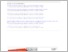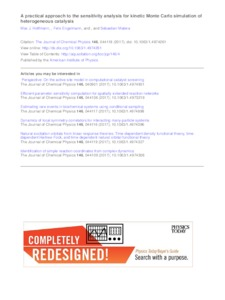Repository: Freie Universität Berlin, Math Department

# A practical approach to the sensitivity analysis for kinetic Monte Carlo simulation of heterogeneous catalysis

Hoffmann, M. J. and Engelmann, F. and Matera, S. (2017) A practical approach to the sensitivity analysis for kinetic Monte Carlo simulation of heterogeneous catalysis. The Journal of Chemical Physics, 146 (044118). ISSN 00219606Preview

672kB

## Abstract

Lattice kinetic Monte Carlo simulations have become a vital tool for predictive quality atomistic understanding of complex surface chemical reaction kinetics over a wide range of reaction conditions. In order to expand their practical value in terms of giving guidelines for the atomic level design of catalytic systems, it is very desirable to readily evaluate a sensitivity analysis for a given model. The result of such a sensitivity analysis quantitatively expresses the dependency of the turnover frequency, being the main output variable, on the rate constants entering the model. In the past, the application of sensitivity analysis, such as degree of rate control, has been hampered by its exuberant computational effort required to accurately sample numerical derivatives of a property that is obtained from a stochastic simulation method. In this study, we present an efficient and robust three-stage approach that is capable of reliably evaluating the sensitivity measures for stiff microkinetic models as we demonstrate using the CO oxidation on RuO2 (110) as a prototypical reaction. In the first step, we utilize the Fisher information matrix for filtering out elementary processes which only yield negligible sensitivity. Then we employ an estimator based on the linear response theory for calculating the sensitivity measure for non-critical conditions which covers the majority of cases. Finally, we adapt a method for sampling coupled finite differences for evaluating the sensitivity measure for lattice based models. This allows for an efficient evaluation even in critical regions near a second order phase transition that are hitherto difficult to control. The combined approach leads to significant computational savings over straightforward numerical derivatives and should aid in accelerating the nano-scale design of heterogeneous catalysts.

Item Type: Article Mathematical and Computer Sciences > Mathematics > Applied Mathematics Department of Mathematics and Computer Science > Institute of Mathematics > Geophysical Fluid Dynamics Group 2098 Ulrike Eickers 22 Aug 2017 11:02 22 Aug 2017 11:02

Repository Staff Only: item control page